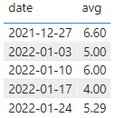cancel
Showing results for
Did you mean:Helper III

## 4 week rolling average

Hi, I need to be able to create a 4 week rolling average off of calendar weeks from Monday start to Sunday end.

So if I opened the report on a Wed I need to make sure the calculation cuts off on Sunday before.

This is an example of what I would like to acheive - the resulting average line. It would be great to make the whole calculation in one measure so I don't have to make 4 and average the 4 after - would be less measures to make:

 Week Start Week End Sales 27/12/21 2/1/2022 100 3/1/2022 9/1/2022 121 10/1/2022 16/1/2022 123 17/1/2022 23/1/2022 122 AVERAGE 116.5
8 REPLIES 8Community Support

Hi  @jnrezk ,

I created some data:Here are the steps you can follow：

1. Create calculated column.

``week = WEEKNUM('Table'[date],2)``
``weekday = WEEKDAY('Table'[date],2)``
``````week_column =
var _lastweek=CALCULATE(MAX('Table'[week]),FILTER(ALL('Table'),YEAR('Table'[date])=YEAR(EARLIER('Table'[date]))))
return
IF([week]=_lastweek&&WEEKDAY(DATE(YEAR([date]),12,31),2)<>7,1,[week])``````2. Create measure.

``avg = CALCULATE(AVERAGE('Table'[value]),FILTER(ALL('Table'),'Table'[week_column]=MAX('Table'[week_column])&&YEAR('Table'[date])=YEAR(MAX('Table'[date]))))``
``````min =
var _todayweek=WEEKNUM(TODAY(),1)
return
CALCULATE(MIN('Table'[date]),FILTER(ALL('Table'),'Table'[week_column]=MAX('Table'[week_column])&&'Table'[week_column]>=_todayweek-4&&'Table'[week_column]<=_todayweek))``````
``````Flag =
IF(
MAX('Table'[date])=[min],1,0)``````

3. Place [Flag]in Filters, set is=1, apply filter.4. Result:Best Regards,

Liu Yang

If this post helps, then please consider Accept it as the solution to help the other members find it more quicklyHelper III

@v-yangliu-msft  this seems to be a average daily but I am looking for total weeksSuper User

@jnrezk , Create a date table or week table with rank on week start date

new columns
Week Start date = 'Date'[Date]+-1*WEEKDAY('Date'[Date],2)+1
Week End date = 'Date'[Date]+ 7-1*WEEKDAY('Date'[Date],2)
Week Rank = RANKX(all('Date'),'Date'[Week Start date],,ASC,Dense)
OR
Week Rank = RANKX(all('Date'),'Date'[Year Week],,ASC,Dense) //YYYYWW format

Then try measures like

Last 4 weeks = CALCULATE(sum('Table'[Qty]), FILTER(ALL('Date'),'Date'[Week Rank]>=max('Date'[Week Rank])-5 && 'Date'[Week Rank]<=max('Date'[Week Rank]) -1 ))

!! Microsoft Fabric !!
Microsoft Power BI Learning Resources, 2023 !!
Learn Power BI - Full Course with Dec-2022, with Window, Index, Offset, 100+ Topics !!
Did I answer your question? Mark my post as a solution! Appreciate your Kudos !! Proud to be a Super User! !!Helper III

@amitchandak Thanks for this. I have created a few new columns to calendar including Week Year and Last 4 Weeks as you said - the numbers don't seem to be correct ... i attached an example and hoped you could please alter it or let me know what I did wrong? Thanks!!https://drive.google.com/file/d/1pFO1IL-Pt9UWqlvd5j2qmLuNW4Q-Vzlz/view?usp=sharingSuper User

@jnrezk , You need to create measures for rolling

Avg = CALCULATE(AverageX(values('Date'[Week Rank]), calculate(sum('Table'[Qty])) ), FILTER(ALL('Date'),'Date'[Week Rank]>=max('Date'[Week Rank])-5 && 'Date'[Week Rank]<=max('Date'[Week Rank]) -1 ))

a new column will be like

CALCULATE(sum('Table'[Qty]), FILTER(('Table'),'Table'[Week Rank]>=earlier('Table'[Week Rank])-5 && 'Table'[Week Rank]<=earlier('Table'[Week Rank]) -1 ))

!! Microsoft Fabric !!
Microsoft Power BI Learning Resources, 2023 !!
Learn Power BI - Full Course with Dec-2022, with Window, Index, Offset, 100+ Topics !!
Did I answer your question? Mark my post as a solution! Appreciate your Kudos !! Proud to be a Super User! !!Helper III

@amitchandak This is what I already did - can you check my file I linked and let me know what adjustments I should make?Super User

!! Microsoft Fabric !!
Microsoft Power BI Learning Resources, 2023 !!
Learn Power BI - Full Course with Dec-2022, with Window, Index, Offset, 100+ Topics !!
Did I answer your question? Mark my post as a solution! Appreciate your Kudos !! Proud to be a Super User! !!Helper III

Hi, @amitchandak I gave access - do you have it now?